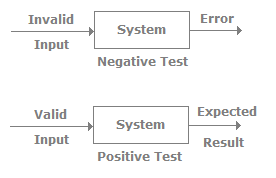# Testing Techniques - Software Testing Interview Questions and Answers

## Why "Software Testing - Testing Techniques" Interview Questions?

In this section you can learn and practice interview questions based on ""Software Testing - Testing Techniques"" and improve your skills in order to face the interview, competitive examination and various entrance tests (CAT, GATE, GRE, MAT, Bank Exam, Railway Exam etc.) with full confidence.

## Where can I get "Software Testing - Testing Techniques" interview questions and answers with explanation?

IndiaBIX provides you lots of fully solved "Software Testing - Testing Techniques" interview questions and answers with Explanation. All students, freshers can download "Software Testing - Testing Techniques" quiz questions with answers as PDF files and eBooks. Solved examples with detailed answer description, explanation are given and it would be easy to understand. View the solution for the problems with feel and good user interface; easily go through all questions and answers.

## How to answer "Software Testing - Testing Techniques" interview questions?

You can easily answer the interview questions based on "Software Testing - Testing Techniques" by practicing the exercises given below.

1. Can you explain boundary value analysis?
In some projects there are scenarios where we need to do boundary value testing. For instance, let's say for a bank application you can withdraw a maximum of 25000 and a minimum of 100. So in boundary value testing we only test the exact boundaries rather than hitting in the middle. That means we only test above the max and below the max. This covers all scenarios. The following figure shows the boundary value testing for the bank application which we just described. TC1 and TC2 are sufficient to test all conditions for the bank. TC3 and TC4 are just duplicate/redundant test cases which really do not add any value to the testing. So by applying proper boundary value fundamentals we can avoid duplicate test cases, which do not add value to the testing.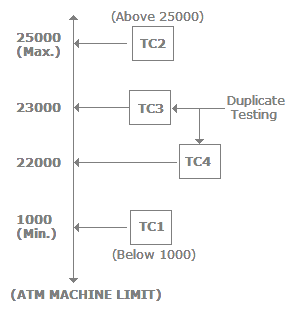2. Can you explain equivalence partitioning?
In equivalence partitioning we identify inputs which are treated by the system in the same way and produce the same results. You can see from the following figure applications TC1 and TC2 give the same results (i.e., TC3 and TC4 both give the same result, Result2). In short, we have two redundant test cases. By applying equivalence partitioning we minimize the redundant test cases.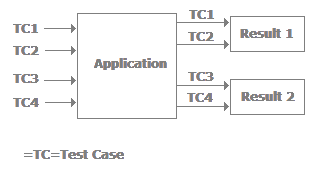So apply the test below to see if it forms an equivalence class or not:
• All the test cases should test the same thing.
• They should produce the same results.
• If one test case catches a bug, then the other should also catch it.
• If one of them does not catch the defect, then the other should not catch it.
3. Can you explain random/monkey testing?
Random testing is sometimes called monkey testing. In Random testing, data is generated randomly often using a tool. For instance, the following figure shows how randomly-generated data is sent to the system. This data is generated either using a tool or some automated mechanism. With this randomly generated input the system is then tested and results are observed accordingly.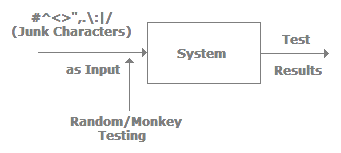Random testing has the following weakness:
• They are not realistic.
• Many of the tests are redundant and unrealistic.
• You will spend more time analyzing results.
• You cannot recreate the test if you do not record what data was used for testing.

This kind of testing is really of no use and is normally performed by newcomers. Its best use is to see if the system will hold up under adverse effects.
4. What are semi-random test cases?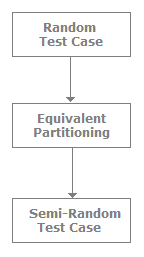As the name specifies semi-random testing is nothing but controlling random testing and removing redundant test cases. So what we do is perform random test cases and equivalence partitioning to those test cases, which in turn removes redundant test cases, thus giving us semi-random test cases.
5. Can you explain a pair-wise defect?
Orthogonal array is a two-dimensional array in which if we choose any two columns in the array and all the combinations of numbers will appear in those columns. The following figure shows a simple L9(34) orthogonal array. In this the number 9 indicates that it has 9 rows. The number 4 indicates that it has 4 columns and 3 indicates that each cell contains a 1, 2, and 3. Choose any two columns. Let's choose column 1 and 2. It has (1,1), (1,2), (1,3), (2,1), (2,2), (2,3), (3,1), (3,2), (3,3) combination values. As you can see these values cover all the values in the array. Compare the values with the combination of column 3 and 4 and they will fall in some pair. This is applied in software testing which helps us eliminate duplicate test cases.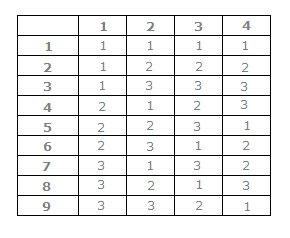6. What is negative and positive testing?
A negative test is when you put in an invalid input and receive errors.

A positive test is when you put in a valid input and expect some action to be completed in accordance with the specification.